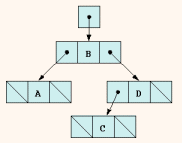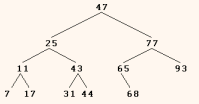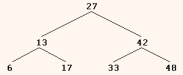Previous Entry Next Entry→

September 16, 2005

There are two very basic types of linked list sub types other than the stack: the queue and the tree.  A queue is the supermarket "First in First Out/FIFO" model and a tree is basically just non-linear with branches but no circuits.  For a queue, the push is the same as "enqueue"  and instead of a pop off the top, a "dequeue" off the bottom.

The CPU of a computer typically needs to ultimately queue the requests made on its processor, in at least one queue.

All of this works precisely as stack did except for the variation of provisions for enqueue and dequeue as compared to push and pop.  No more comment is needed really, so here's the queue.h header and I'll move on to trees.

 // Fig. 17.13: queue.h // Template Queue class definition derived from class List. #ifndef QUEUE_H #define QUEUE_H #include "list.h" // List class definition template< class QUEUETYPE > class Queue: private List< QUEUETYPE > {   public:     // enqueue calls List function insertAtBack     void enqueue( const QUEUETYPE &data ) {         insertAtBack( data );     } // end function enqueue     // dequeue calls List function removeFromFront     bool dequeue( QUEUETYPE &data )  {         return removeFromFront( data );     } // end function dequeue     // isQueueEmpty calls List function isEmpty     bool isQueueEmpty() const  {         return isEmpty();     } // end function isQueueEmpty     // printQueue calls List function print     void printQueue() const  {         print();     } // end function printQueue     }; // end class Queue #endif
 Unlike queues and stacks, a tree a is nonlinear and two-dimensional data structure. Tree nodes must contain at least two links. Binary trees are trees whose nodes all contain two links (none, one or both of which may be null).  In the diagram at right,  the root node (node B) is the first node in a tree. Each link in the root node refers to a child (nodes A and D). The left child (node A) is the root node of the left subtree (which contains only node A), and the right child (node D) is the root node of the right subtree (which contains nodes D and C). The children of a single node are called siblings (e.g., A and D ). A node with no children is a leaf node (e.g., nodes A and C). Given how these diagrams branch downwards, you might expect them to be called "root" structures.A binary search tree (with no duplicate node values) has the characteristic that the values in any left subtree are less than the value in its parent node, and the values in any right subtree are greater than the value in its parent node. The tree at right illustrates a binary search tree with 12 values. Note that the shape of the binary search tree that corresponds to a set of data can vary, depending on the order in which the values are inserted into the tree7-11-17-25-31-43-44-47-65-68 -77  -  93

In the program of example at the end of Deitel, chapter 17, a binary search tree is created and traversed in three ways—using recursive inorder, preorder and postorder traversals.

The driver program begins by instantiating integer tree intTree of type Tree<int>.  The program prompts for 10 integers, each of which is inserted in the binary tree by calling insertNode.  The program then performs preorder, inorder and postorder traversals (these are explained shortly) of intTree. The program then instantiates floating-point tree doubleTree of type Tree<double>. The program prompts for 10 double values, each of which is inserted in the binary tree by calling insertNode. The program then performs preorder, inorder and postorder traversals of doubleTree.

class template declares as its friend the Tree class template, and provides pointers to left and right subtrees as private member data.   The constructor sets data to the value supplied as a constructor argument and sets pointers leftPtr and rightPtr to zero (thus initializing this node to be a leaf node). Member function getData returns the data value.

The Tree class template has private data rootPtr, a pointer to the root node of the tree. The public member functions insertNode (that inserts a new node in the tree) and preorderTraversal, inorderTraversal and postorderTraversal, each of which calls its own separate recursive utility function to perform the appropriate operations on the internal representation of the tree. The Tree constructor initializes rootPtr to zero to indicate that the tree is initially empty.

Tree’s utility function is called by insertNode to recursively insert a node into the tree.  In a binary search tree, nodes can only be inserted as a leaf node.  If the tree is empty, a new TreeNode is created, initialized and inserted in the tree.

If the tree is not empty, the program compares the value to be inserted with the data value in the root node. If the insert value is smaller, the program recursively calls to insert the value in the left subtree. If the insert value is larger, the program recursively calls to insert the value in the right subtree. If the value to be inserted is identical to the data value in the root node, the program prints the message " dup" and returns without inserting the duplicate value into the tree. Note that insertNode passes the address of rootPtr to   so it can modify the value stored in rootPtr (i.e., the address of the root node). To receive a pointer to rootPtr (which is also a pointer), ’s first argument is declared as a pointer to a pointer to a TreeNode.

 Each of the member functions , and traverses the tree and prints the node values. For the purpose of the following discussion, we use the binary search tree shown here:Function invokes utility function inOrderHelper to perform the inorder traversal of the binary tree. The steps for an inorder traversal are: Traverse the left subtree with an inorder traversal. (This is performed by the call to inOrderHelper.) Process the value in the node—i.e., print the node value. Traverse the right subtree with an inorder traversal. (This is performed by the call to inOrderHelper.)

The value in a node is not processed until the values in its left subtree are processed, because each call to inOrderHelper immediately calls inOrderHelper again with the pointer to the left subtree. The inorder traversal of the tree in Fig. is

6 13 17 27 33 42 48

Note that the inorder traversal of a binary search tree prints the node values in ascending order. The process of creating a binary search tree actually sorts the data—thus, this process is called the binary tree sort.

Function preOrderTraversal invokes utility function preOrderHelper to perform the preorder traversal of the binary tree. The steps for an preorder traversal are:

1. Process the value in the node

2. Traverse the left subtree with a preorder traversal. (This is performed by the call to preOrderHelper

3. Traverse the right subtree with a preorder traversal. (This is performed by the call to preOrderHelperThe value in each node is processed as the node is visited. After the value in a given node is processed, the values in the left subtree are processed. Then the values in the right subtree are processed. The preorder traversal of the tree in Fig. is

`27 13 6 17 42 33 48`

Function postOrderTraversal invokes utility function postOrderHelper to perform the postorder traversal of the binary tree. The steps for an postorder traversal are:

1. Traverse the left subtree with a postorder traversal. (This is performed by the call to postOrderHelper

2. Traverse the right subtree with a postorder traversal. (This is performed by the call to postOrderHelper

3. Process the value in the node

The value in each node is not printed until the values of its children are printed. The postOrderTraversal of the tree diagrammed above is

6 17 13 33 48 42 27

The binary search tree facilitates duplicate elimination. As the tree is being created, an attempt to insert a duplicate value will be recognized, because a duplicate will follow the same “go left” or “go right” decisions on each comparison as the original value did when it was inserted in the tree. Thus, the duplicate will eventually be compared with a node containing the same value. The duplicate value may be discarded at this point.

Searching a binary tree for a value that matches a key value is also fast. If the tree is balanced, then each level contains about twice as many elements as the previous level. So a binary search tree with n elements would have a maximum of log2n levels; thus, a maximum of log2n comparisons would have to be made either to find a match or to determine that no match exists. This means, for example, that when searching a (balanced) 1000-element binary search tree, no more than 10 comparisons need to be made because 210 > 1000. When searching a (balanced) 1,000,000-element binary search tree, no more than 20 comparisons need to be made because 220 > 1,000,000.

 // Fig. 17.17: treenode.h // Template TreeNode class definition. #ifndef TREENODE_H #define TREENODE_H // forward declaration of class Tree template< class NODETYPE > class Tree; template< class NODETYPE > class TreeNode {    friend class Tree< NODETYPE >;    public:       // constructor       TreeNode( const NODETYPE &d )                 : leftPtr( 0 ),                   data( d ),                   rightPtr( 0 )       {          // empty body       } // end TreeNode constructor       // return copy of node's data       NODETYPE getData() const       {          return data;       } // end getData function    private:       TreeNode< NODETYPE > *leftPtr; // pointer to left subtree       NODETYPE data;       TreeNode< NODETYPE > *rightPtr; // pointer to right subtree }; // end class TreeNode #endif

 // Fig. 17.18: tree.h // Template Tree class definition. #ifndef TREE_H #define TREE_H #include using std::endl; #include #include "treenode.h" template< class NODETYPE > class Tree {    public:       Tree();       void insertNode( const NODETYPE & );       void preOrderTraversal() const;       void inOrderTraversal() const;       void postOrderTraversal() const;    private:       TreeNode< NODETYPE > *rootPtr;       // utility functions       void insertNodeHelper(               TreeNode< NODETYPE > **, const NODETYPE & );       void preOrderHelper( TreeNode< NODETYPE > * ) const;       void inOrderHelper( TreeNode< NODETYPE > * ) const;       void postOrderHelper( TreeNode< NODETYPE > * ) const; }; // end class Tree // constructor template< class NODETYPE > Tree< NODETYPE >::Tree()  {    rootPtr = 0; } // end Tree constructor // insert node in Tree template< class NODETYPE > void Tree< NODETYPE >::insertNode( const NODETYPE &value ) {    insertNodeHelper( &rootPtr, value ); } // end function insertNode // utility function called by insertNode; receives a pointer // to a pointer so that the function can modify pointer's value template< class NODETYPE > void Tree< NODETYPE >::insertNodeHelper(               TreeNode< NODETYPE > **ptr, const NODETYPE &value ) {    // subtree is empty; create new TreeNode containing value    if ( *ptr == 0 )       *ptr = new TreeNode< NODETYPE >( value );    else // subtree is not empty        // and data to insert is less than data in current node    if ( value < ( *ptr )->data )       // then go ahead and insert the data to the left       // Note that this is a recursive call.       insertNodeHelper( &( ( *ptr )->leftPtr ), value );    else    // data to insert is greater than data in current node    if ( value > ( *ptr )->data )       // otherwise, insert data to the right       insertNodeHelper( &( ( *ptr )->rightPtr ), value );    else // unless duplicate data, in which case...skip it!       cout << value << " dup" << endl; } // end function insertNodeHelper // begin preorder traversal of Tree template< class NODETYPE > void Tree< NODETYPE >::preOrderTraversal() const  {    preOrderHelper( rootPtr ); } // end function preOrderTraversal // utility function to perform preorder traversal of Tree template< class NODETYPE > void Tree< NODETYPE >::preOrderHelper(                           TreeNode< NODETYPE > *ptr ) const  {    if ( ptr != 0 ) {       cout << ptr->data << ' '; // process node       preOrderHelper( ptr->leftPtr ); // go to left subtree       preOrderHelper( ptr->rightPtr ); // go to right subtree    } // end if } // end function preOrderHelper // begin inorder traversal of Tree template< class NODETYPE > void Tree< NODETYPE >::inOrderTraversal() const {    inOrderHelper( rootPtr ); } // end function inOrderTraversal // utility function to perform inorder traversal of Tree template< class NODETYPE > void Tree< NODETYPE >::inOrderHelper(                         TreeNode< NODETYPE > *ptr ) const  {    if ( ptr != 0 ) {       inOrderHelper( ptr->leftPtr ); // go to left subtree       cout << ptr->data << ' '; // process node       inOrderHelper( ptr->rightPtr ); // go to right subtree    } // end if } // end function inOrderHelper // begin postorder traversal of Tree template< class NODETYPE > void Tree< NODETYPE >::postOrderTraversal() const {    postOrderHelper( rootPtr ); } // end function postOrderTraversal // utility function to perform postorder traversal of Tree template< class NODETYPE > void Tree< NODETYPE >::postOrderHelper(             TreeNode< NODETYPE > *ptr ) const  {    if ( ptr != 0 ) {       postOrderHelper( ptr->leftPtr ); // go to left subtree       postOrderHelper( ptr->rightPtr ); // go to right subtree       cout << ptr->data << ' '; // process node    } // end if } // end function postOrderHelper #endif

 // Fig. 17.19: fig17_19.cpp // Tree class test program. #include using std::cout; using std::cin; using std::fixed; #include using std::setprecision; #include "tree.h" // Tree class definition int main() {    Tree< int > intTree; // create Tree of int values    int intValue;    cout << "Enter 10 integer values:\n";    for( int i = 0; i < 10; i++ ) {       cin >> intValue;       intTree.insertNode( intValue );    } // end for    cout << "\nPreorder traversal\n";    intTree.preOrderTraversal();    cout << "\nInorder traversal\n";    intTree.inOrderTraversal();    cout << "\nPostorder traversal\n";    intTree.postOrderTraversal();    Tree< double > doubleTree; // create Tree of double values    double doubleValue;    cout << fixed << setprecision( 1 )          << "\n\n\nEnter 10 double values:\n";    for ( int j = 0; j < 10; j++ ) {       cin >> doubleValue;       doubleTree.insertNode( doubleValue );    } // end for    cout << "\nPreorder traversal\n";    doubleTree.preOrderTraversal();    cout << "\nInorder traversal\n";    doubleTree.inOrderTraversal();    cout << "\nPostorder traversal\n";    doubleTree.postOrderTraversal();    cout << endl;    char gottto;    cin>> gottto;    return 0; }  // end main